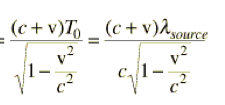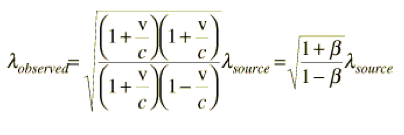# Calculating the doppler red shift

## Homework Statement

I'm working on this problem here:

http://hyperphysics.phy-astr.gsu.edu/hbase/astro/redshf2.html

The answer is already listed, it's the steps I'm worried about.I don't see how they get from step 1 to step 2 here. They don't even tell me what T is. It seems that they are substitution T into the equation. I would think T = lambda/c+v but that's not what they're doing here.I can't get from step 2 to 3, nor 3 to 4. They don't tell you what the equation for lambda source is, so I don't see how they work that into the equation.

Beta = v/c

Well if that's true then (1+v/c)^2 = 1 + 2v/c + (v/c)^2 not what they have.

## Answers and Replies

Hi Robertjford80
T is the period of oscillation, so you have λ=cT or T=λ/c (since the propagation speed of the wave is the speed of light)
This is the substitution made in the first step to the second.
To go to the next step, you use a simple identity
1-x^2=(1-x)(1+x) (for the uppur part, the square comes from puttint everything in the square root)
The next step is just simplifying by (1+v/c) and defining β.

Cheers...

if T = lamba/c and if lambda = c/v then (c/v)/c = 1/v. I don't see that in the equation. I can see how they go from 1-x^2 to (1-x)(1+x) in the denominator but not in the numerator.

I understand step 3 to 4 now.

T=λ/c so the T in the first step is simply replaced by it.
Look at the two equations, there is nothing else to it, you replace T by λ/c, and the c goes down
This c is later what goes in the numerator:
(c+v)/c = 1+v/c
you square it to put it into the square root, and it becomes
√[(1+v/c)(1+v/c)]

Cheers...

I understand now. thanks.